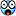# Thread: Failed test question (This is fun)

1. ## Failed test question (This is fun)

A mate of mine had to do a test recently for a job. He gave me one of the questions and for the life of me it makes no sense.
This is the question :
Code:
```A sequence of numbers is called arithmetic if it consists of at least three elements and if the difference between any two consecutive elements
is the same. For example, these are arithmetic sequences:

1,  3,  5,  7, 9
7,  7,  7,  7
3, -1, -5, -9

The sequence [1, 1, 2, 5, 7] is not arithmetic.

A zero-indexed array A consisting of N numbers is given.
A slice of that array is any pair of integers (P, Q) such that 0 ≤ P < Q < N.
A slice (P, Q) of array A is called arithmetic if the sequence:

A[P], A[P+1], ..., A[Q−1], A[Q]
is arithmetic. In particular, this means that P + 1 < Q.
Write a function:
class Solution { int solution(int[] A); }
that, given array A consisting of N numbers, returns the number of arithmetic slices in A. The function should return −1 if the result exceeds 1,000,000,000.
For example, given array A such that:
A = -1
A =  1
A =  3
A =  3
A =  3
A =  2
A =  1
A =  0
the function should return 5 because there are five arithmetic slices of that array, namely:
(0, 2)  (2, 4)  (4, 6)  (4, 7)  (5, 7)
Assume that:
N is an integer within the range [0..100,000];
each element of array A is an integer within the range [−2,147,483,648..2,147,483,647].
Complexity:
expected worst-case time complexity is O(N);
expected worst-case space complexity is O(N), beyond input storage (not counting the storage required for input arguments).
Elements of input arrays can be modified.```I don't get this :
Code:
```A slice of that array is any pair of integers (P, Q) such that 0 ≤ P < Q < N.
A slice (P, Q) of array A is called arithmetic if the sequence:

A[P], A[P+1], ..., A[Q−1], A[Q]
is arithmetic. In particular, this means that P + 1 < Q.```
Can anyone explain so I don't always feel so stupid?Reply With Quote

2. ## Re: Failed test question (This is fun)

P and Q are indexes in the array. If an array has 10 elements, indexed 0 to 9, then one slice is from index 4 to 7, so P is 4 and Q is 7. Let's say that the array is:
Code:
`8, 18, 2, 3, 5, 7, 9, 11, 2, 8`
then that array is not arithmetic but the elements from index 4 to 7 are:
Code:
`5, 7, 9, 11`
and that slice is arithmetic.Reply With Quote

####Posting Permissions

• You may not post new threads
• You may not post replies
• You may not post attachments
• You may not edit your posts
•

Featured